##### Search### 1. SECTION I (50 Marks)

ANSWER ALL QUESTIONS IN THIS SECTION
1.

a) Write the odd numbers in descending order between 1 and 10 inclusive. (1mk)

b) Round-off the number formed to 3 significant figures. (1mk)

c) Find the total value of digit 7 in the new number in 1(b) above. (2mks)

4 marks

2.

The L.C.M of three numbers is 360 and the G.C.D of the same numbers is 2. If one of the numbers is 40. Find the other two numbers. (3mk

4 marks

3.

Evaluate ;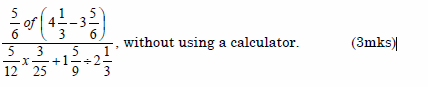3 marks

4.

4. Solve for x in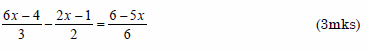3 marks

5.

Simplify completely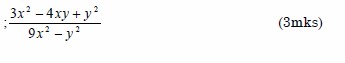3 marks

6.

Use square root and reciprocal tables only to evaluate;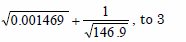decimal places. (3mks)

3 marks

7.

Given that Q is an acute angle and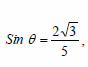find without using calculators or mathematical tables, tan(90-Q)0. (4mks)

4 marks

8.

Find the integral values of x which satisfy the following inequality.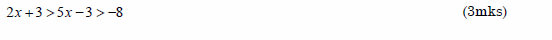3 marks

9.

A surveyor recorded the information about a tea farm in his field book as in the table below.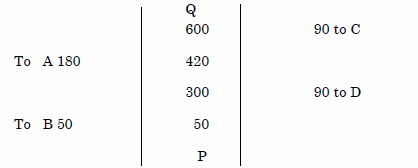a) Given that PQ=650m, make a sketch of the field. (2mks)

b) Hence find the area of the field in hectares. (2mks)

3 marks

10.

A two digit number is such that the sum of its digits is 13. The product of twice the tens digit and the units digit minus the original number is 22. Find the number. (3mks)

3 marks

11.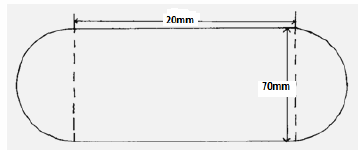The above figure(not drawn to scale) shows the cross-section of a metal bar of
length 3 meters. The ends are equal semi-circles. Determine the mass of the
metal bar in kilograms if the density of the metal is 8.87g/cm3. (3mks)

3 marks

12.

In 2007 parliamentary election in Bumula constituency, only 55% of the voters in
(3mks)

3 marks

13.

Solve for n in the equation.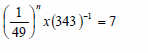(3mks)

3 marks

14.

Evaluate without using tables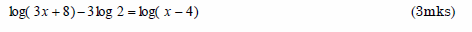3 marks

15.

Given the ratio x:y=2:3, find the ratio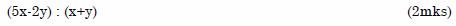2 marks

16.

The diagram below shows a square garden surrounded by a path 2m wide. Given
that the area of the plot of land is 784m2
Find the area of the flower garden. (3mks)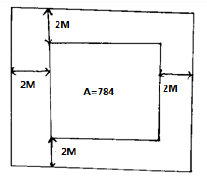3 marks

### 2. SECTION II (50 Marks)

Answer only five questions in this section in the spaces provided.
17.

In the figure below, ROT is a diameter. Angles QTP=480, TQR=760 and SRT=370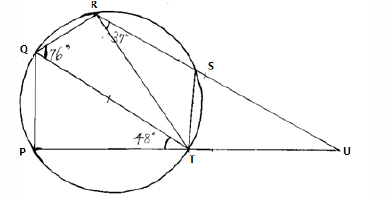Giving reasons, find
a) Angle RST (2mks)

b) Angle SUT (2mks)

c) Angle ROT (2mks)

d) Angle PST (2mks)

e) Angle POS (2mks)

10 marks

18.

a) The points A1(-3,0) and B1(-1,0) are images of A(6,6) and B(2,6) under an enlargement. Calculate the centre of enlargement and the linear scale factor. (4mks)

b) A model of a freezer has a rectangular shutter measuring 4cm by 2cm. The area of the actual shutter of the freezer is 0.72m2
i) Given that the actual freezer is 2.7m long, calculate the length of the model in centimeters. (3mks)

ii) Calculate the volume in m3 of the actual freezer if the models’ volume is 12cm3 (3mks)

10 marks

19.

19. a) Complete the table below for the values of x from -3 to 4; for the function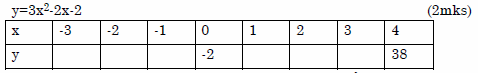c) use the graph in (b) to solve the equations: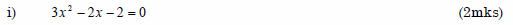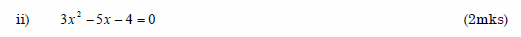10 marks

20.

The figure below shows two circles with centres at A and B. The circles intersect at P and R. AP=8cm, BP=6cm and angle PBR=600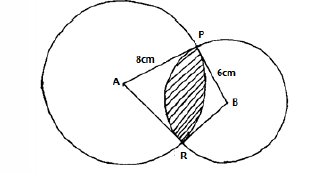a) Calculate
i) The length of PR (2mks)

ii) The angle PAR (3mks)

iii) Hence or otherwise find the shaded area. (5mks)

10 marks

21.

. A trader sold an article at sh.4,800 after allowing his customer a 12% discount on/ the marked price of the article. In so doing he made a profit of 45%.
a) Calculate
i) The marked price of the article. (3mks)

ii) The price at which the trader had bought the article. (2mks)

b) If the trader had sold the same article without giving a discount, calculate the percentage profit he would have made. (3mks)

c) To clear his stock, the trader decided to sell the remaining articles at a loss of 12.5%. Calculate the price at which he sold each article. (2mks)

10 marks

22.

Three quantities P,Q, and R are such that P varies directly as the cube of Q and inversely as the square of R.
a) Given that P=16 when Q=2 and R= 3. Determine the value of R when P=288 and Q=4. (5mks)

b) Q decreases by 30% while R increases by 40%. Find the percentage decrease or increase in P. (5mks)

10 marks

23.

Two airports A and B are such that B is 500km due East of A. Two planes P and Q take off from A and B respectively and at the same time. Plane P flies at 360km/h on a bearing of 0300. Plane Q flies at 240km/h on a bearing of 3150. The two planes land after 90 minutes. Using a scale of 1:10 000 000.
a) Show the positions of the planes after 90minutes. (7mks)

b) Find the distance between the planes after 90 minutes (1mk)

c) Find:
i) The bearing of plane P from plane Q. (1mk)

ii) The bearing of plane Q from plane P after 90 minutes. (1mk)

10 marks

24.

The diagram below shows a histogram representing marks obtained in a certain test.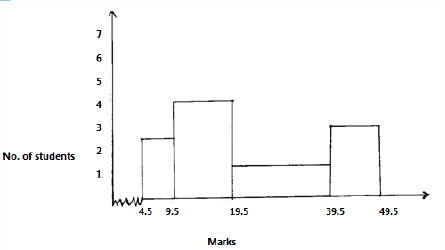a) Prepare a frequency distribution table for the data. (3mks)

b) Estimate
i) The mean mark. (5mks)

ii) The median mark. (2mks)

10 marks

Back Top